Question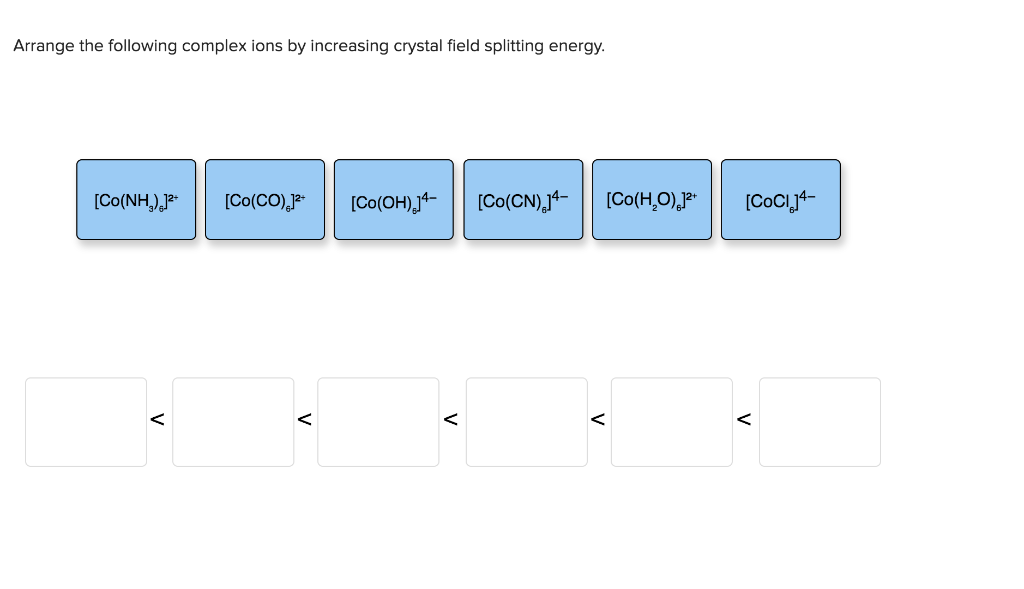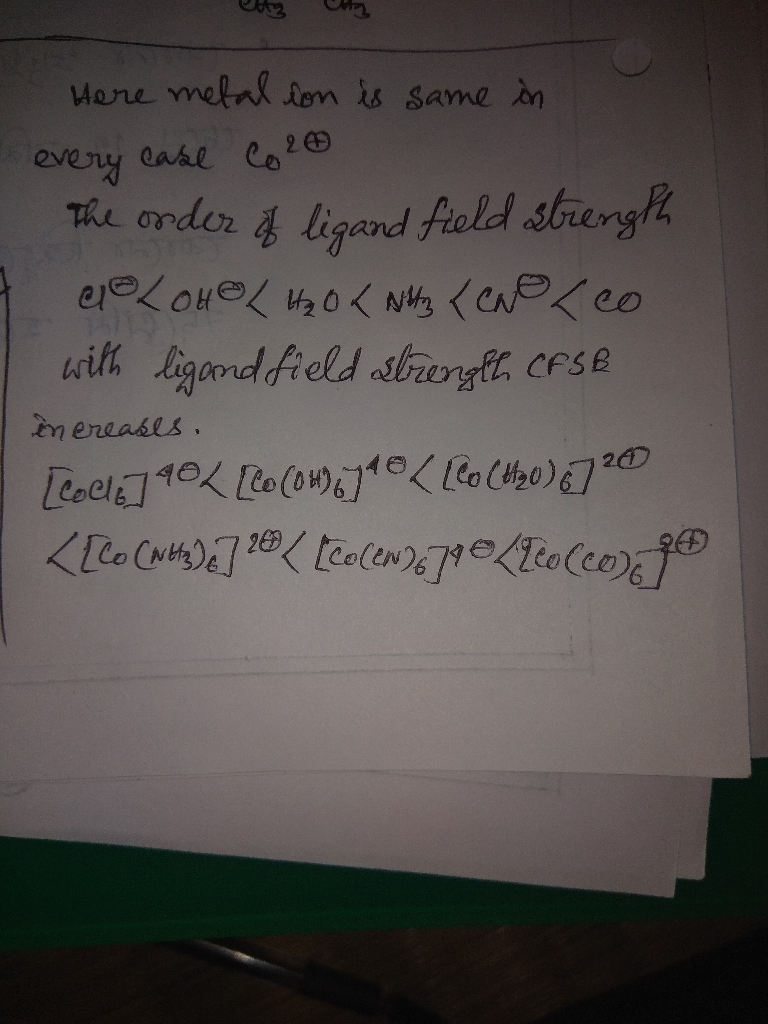#### Earn Coins

Coins can be redeemed for fabulous gifts.

Similar Homework Help Questions
• ### Calculate the crystal field stabilization energy valiue for the complex Cr(NH)J*. The crystal field splitting energy...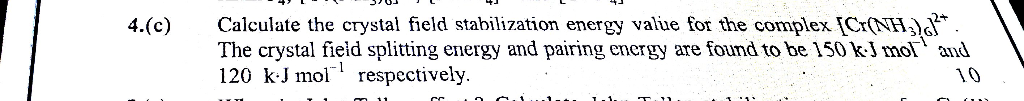Calculate the crystal field stabilization energy valiue for the complex Cr(NH)J*. The crystal field splitting energy and pairing energy are found to be 150 kJ mol and 120 kJ mol respectively. 4.(c) 10 cc. Calculate the crystal field stabilization energy valiue for the complex Cr(NH)J*. The crystal field splitting energy and pairing energy are found to be 150 kJ mol and 120 kJ mol respectively. 4.(c) 10 cc.

• ### (8 pts) 13. a. Give the crystal field splitting diagram for the complex ion, [Cr(CN)! with...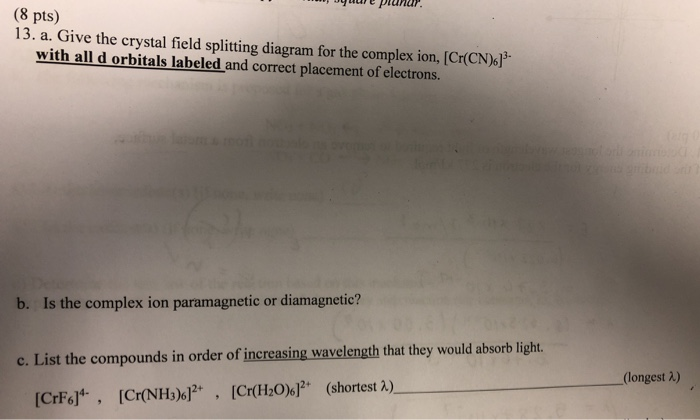(8 pts) 13. a. Give the crystal field splitting diagram for the complex ion, [Cr(CN)! with all d orbitals labeled and correct placement of electrons. b. Is the complex ion paramagnetic or diamagnetic? c. List the compounds in order of increasing wavelength that they would absorb light. (longest 2) (shortest A) [Cr(H2O)6]2. [CMNHJs产 [CrF6]4-, ,

• ### What is the crystal field energy level diagram for the complex [Co(CN).13-? d1 라리라 , 씀씀을...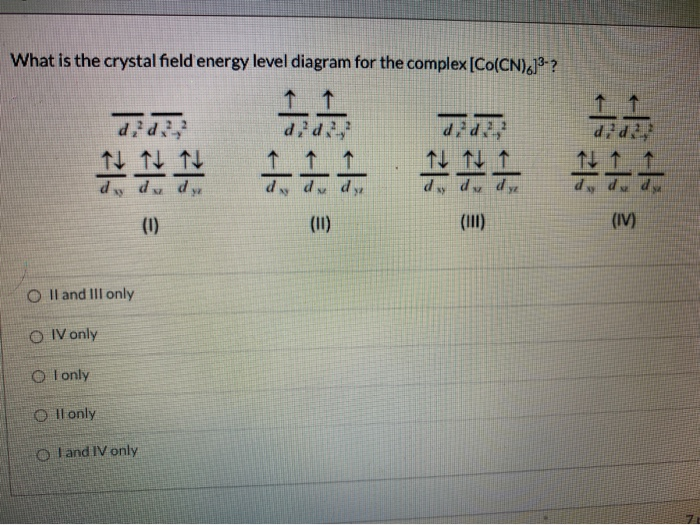What is the crystal field energy level diagram for the complex [Co(CN).13-? d1 라리라 , 씀씀을 은은 (1) (IV) O II and III only OIV only O Tonly O ll only o I and IV only

• ### Date: 1117 mework 10 - Ch. 21-23 A) CN B)NO c)r D) CO Name: E ) en 24. Place the ligands, CN-, Br., H2O, and OH-in o...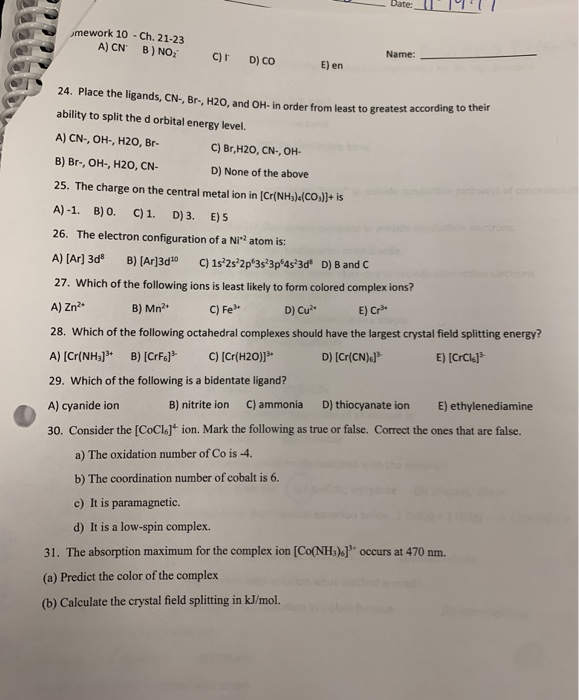Date: 1117 mework 10 - Ch. 21-23 A) CN B)NO c)r D) CO Name: E ) en 24. Place the ligands, CN-, Br., H2O, and OH-in order from least to greatest according ability to split the d orbital energy level. A) CN-, OH, H2O, Br- C) Br,H20, CN-, OH- B) Br., OH, H2O, CN- D) None of the above 25. The charge on the central metal ion in (Cr(NH3)(CO3)2+ is A)-1. B) 0. C) 1. D) 3. E) 5 26....

• ### 5. The complex [Cu(CN).]* undergoes tetragonal elongation due to Jahn-Teller distortion Draw the Crystal field splitting...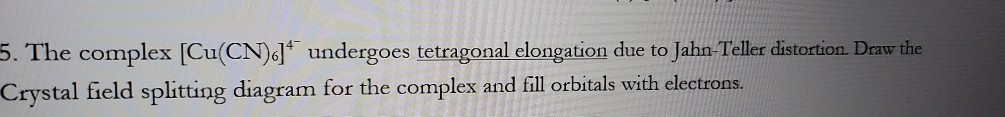5. The complex [Cu(CN).]* undergoes tetragonal elongation due to Jahn-Teller distortion Draw the Crystal field splitting diagram for the complex and fill orbitals with electrons.

• ### Arrange these complexes in order of octahedral splitting energy, A.. Largest A. [Ru(NO2)613- [Cr(OH),13- [Co(NH,613+ [Cr(NO2)612-...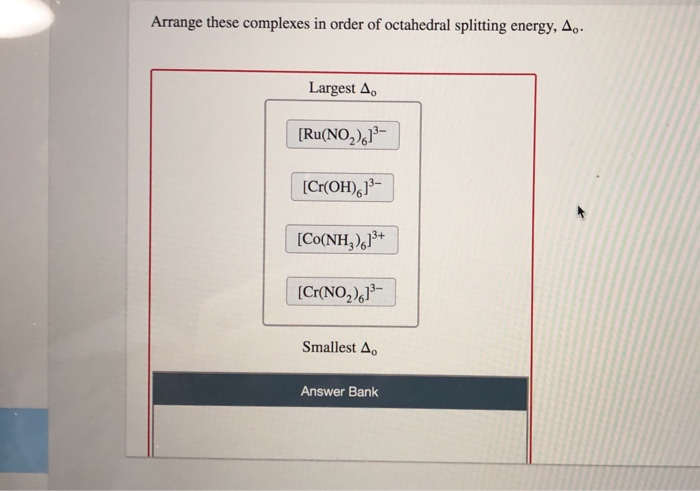Arrange these complexes in order of octahedral splitting energy, A.. Largest A. [Ru(NO2)613- [Cr(OH),13- [Co(NH,613+ [Cr(NO2)612- Smallest A. Answer Bank

• ### 2. a. Draw and show the crystal field splitting of the d orbitals in an octahedral...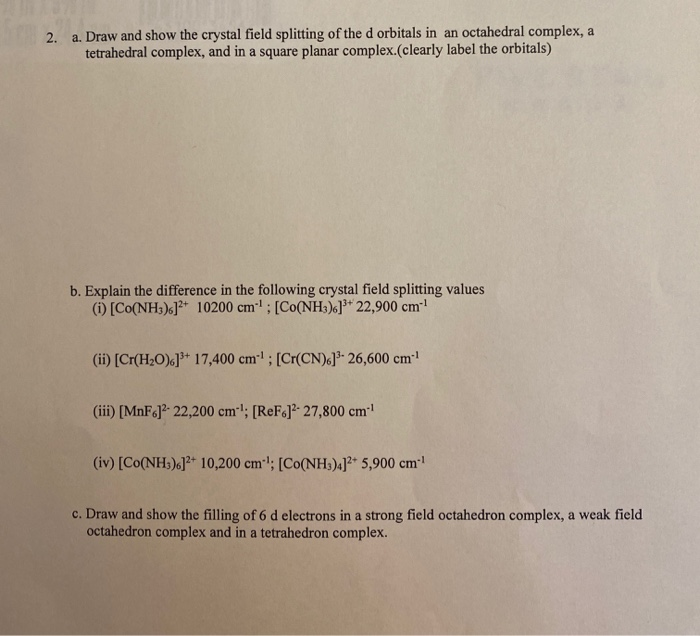2. a. Draw and show the crystal field splitting of the d orbitals in an octahedral complex, a tetrahedral complex, and in a square planar complex.(clearly label the orbitals) b. Explain the difference in the following crystal field splitting values (1) Co(NH3).]2+ 10200 cm"! : [CO(NH3).]* 22,900 cm (ii) [Cr(H20).]** 17,400 cm. ; [Cr(CN).]3-26,600 cm (iii) [MnF6]?- 22,200 cm-"; [ReF.]?- 27,800 cm- (iv) [Co(NH3).]2+ 10,200 cm '; [Co(NH3)4]2+ 5,900 cm c. Draw and show the filling of 6 d electrons...

• ### show all steps please What is the crystal field energy level diagram for the complex (Co(CN).1?...show all steps please What is the crystal field energy level diagram for the complex (Co(CN).1? 11 did! ttt! 1 1 11 ddd dy dud d. de do 2.1 (1) (111) (IV)

• ### What is the crystal field energy level diagram for the complex [Co(CN)613-? 2 did 2 Xy...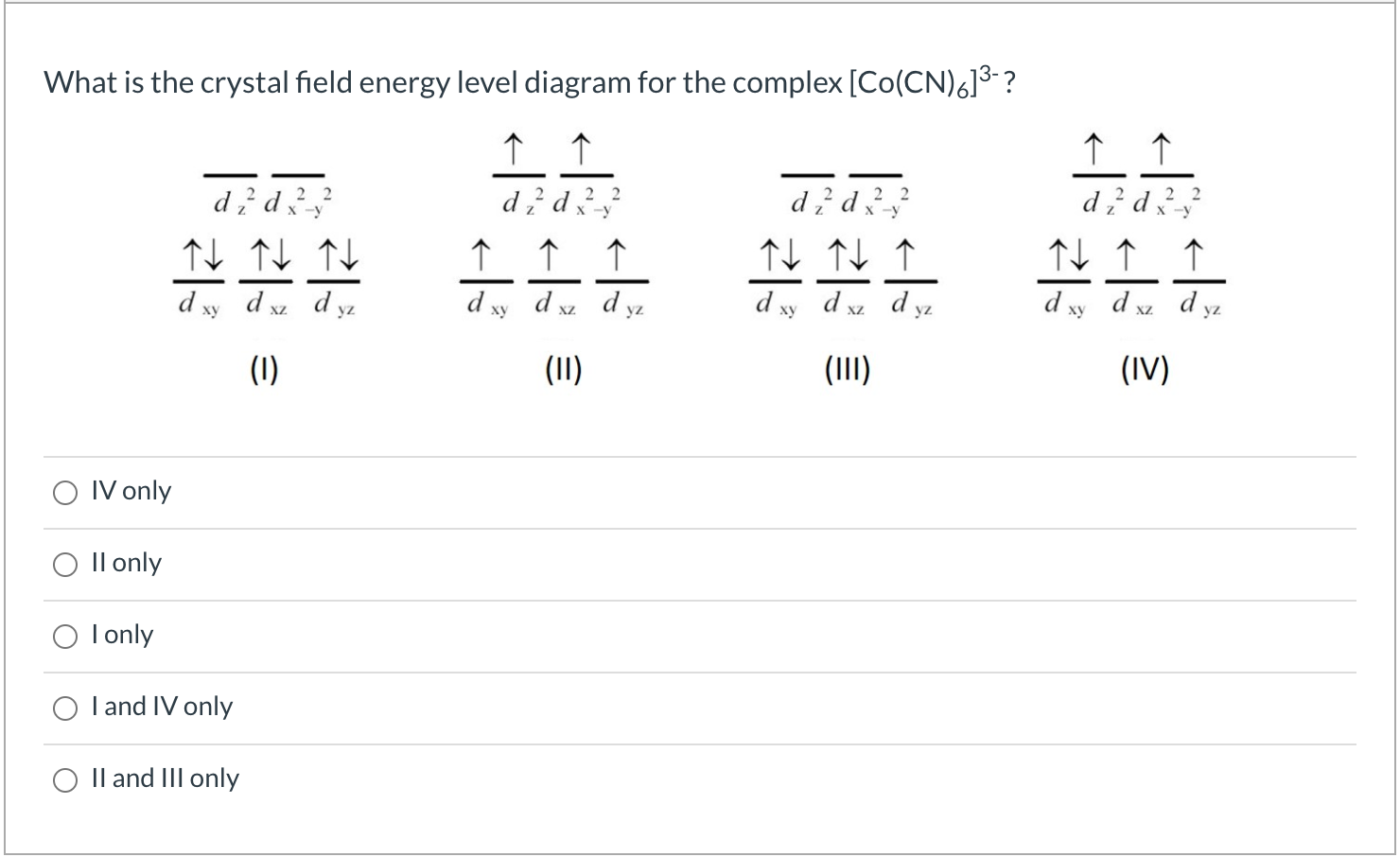What is the crystal field energy level diagram for the complex [Co(CN)613-? 2 did 2 Xy d, ↑ ↑ ↑ d xy d xz dyz 1 1 d?d?? ^ ^ ^ d xv d d, 1 1 1 1 d xy dxz d 1 d d xz dyz dxi ху yz yz (1) (11) (111) (IV) IV only O Il only I only I and IV only II and III only

• ### The complex [Fe(H2O)6]3+ has a maximum absorbance at 724 nm. a.Calculate the crystal field splitting energy,...

The complex [Fe(H2O)6]3+ has a maximum absorbance at 724 nm. a.Calculate the crystal field splitting energy, in kJ, for the complex. b.Predict the colour of the complex.